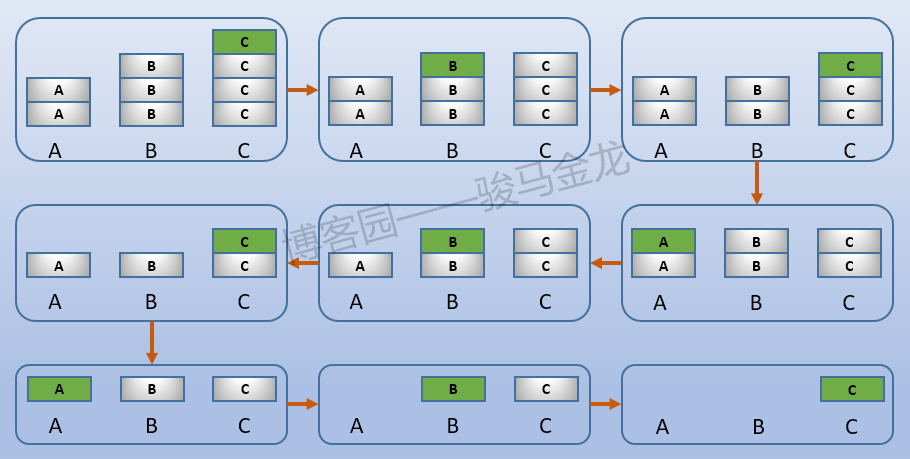# lvs的wrr调度算法

## 1.加权调度算法公式

while (true) {
i = (i + 1) mod n;
if (i == 0) {
cw = cw - gcd(S);
if (cw <= 0) {
cw = max(S);
if (cw == 0)
return NULL;
}
}
if (W(Si) >= cw)
return Si;
}


## 2.加权调度通俗规律

1.先约分
2.从最大权重开始调度
3.同权重的后端，从前向后调度

1. 调度C
调度之后，比率变成A:B:C=2:3:3，B和C权重相同，从B开始调度
2. 调度B
调度之后，比率变成A:B:C=2:2:3，所以下次调度C
3. 调度C
调度之后，比率变成A:B:C=2:2:2，下次从A开始
当权重全部调整到相同值时，就按照先后顺序不断循环，直到调度完所有权重
4. 调度A，调度之后，比率变成A:B:C=1:2:2
5. 调度B，调度之后，比率变成A:B:C=1:1:2
6. 调度C，调度之后，比率变成A:B:C=1:1:1
7. 调度A，调度之后，比率变成A:B:C=0:1:1
8. 调度B，调度之后，比率变成A:B:C=0:0:1
9. 调度C，调度之后，比率变成A:B:C=0:0:0
10. 进入下一个调度循环，顺序是：CBCABCABC1. 调度D
2. 调度C
3. 调度D
4. 调度B
5. 调度C
6. 调度D
7. 调度A
8. 调度B
9. 调度C
10. 调度D

# nginx的wrr调度算法

1. 所有权重加总得到总权重(total)：A+B+C=N
2. 第一个请求进来，选择权重最大的。假设为a，于是选中A。选中之后，对选中的节点的权重减去总权重N，于是现在的比例是a:b:c=A-N:B:C
3. 第二个请求进入，将比如全部加上原始比例(eff_weight)，得到(A-N+A):(B+B):(C+C)，然后选中最大的权重节点，假如选中B。选中之后，对选中的节点的权重减去总权重N，于是比例变为(2A-N):(2B-N):2C
4. 之后每个请求进来，都首先加上原始比例，然后选择权重最大的，并对最大的权重值减去总权重N。
5. 每一轮询的最后一次调度，都会使被选中的节点权重变为N，其它权重变为0，减去总权重之后全部变成0，使得下一轮轮询调度开始，重新回到原始比例。

1. 第1个请求，a权重最大，选中a
• 选中之后，比例变为a:b:c=-5:3:2
2. 第2个请求，加上原始比例，得到a:b:c=-1:6:4，选中b
• 选中之后，比例变为a:b:c=-1:-3:4
3. 第3个请求，加上原始比例，得到a:b:c=3:0:6，选中c
• 选中之后，比例变为a:b:c=3:0:-3
4. 第4个请求，加上原始比例，得到a:b:c=7:3:-1，选中a
• 选中之后，比例变为a:b:c=-2:3:-1
5. 第5个请求，加上原始比例，得到a:b:c=2:6:1，选中b
• 选中之后，比例变为a:b:c=2:-3:1
6. 第6个请求，加上原始比例，得到a:b:c=6:0:3，选中a
• 选中之后，比例变为a:b:c=-3:0:3
7. 第7个请求，加上原始比例，得到a:b:c=1:3:5，选中c
• 选中之后，比例变为a:b:c=1:3:-4
8. 第8个请求，加上原始比例，得到a:b:c=5:6:-2，选中b
• 选中之后，比例变为a:b:c=5:-3:-2
9. 第9个请求，加上原始比例，得到a:b:c=9:0:0，选中a
• 选中之后，比例变为a:b:c=0:0:0

posted @ 2018-08-16 22:49  骏马金龙  阅读(2557)  评论(3编辑  收藏